Decimals in number line

Chapter 8 Class 6 Decimals
Concept wise

For numbers with 1 decimal point like

4.2

We draw it in the number line

Now,

4.2 > 4

&       4.2 < 5

So, we draw number line from 4 to 5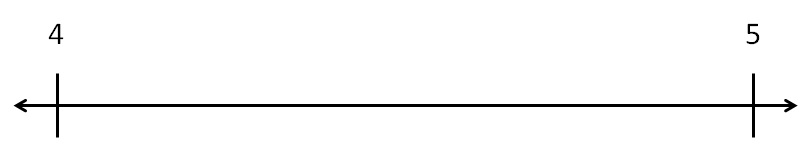We divide number line between 4 & 5 in 10 equal parts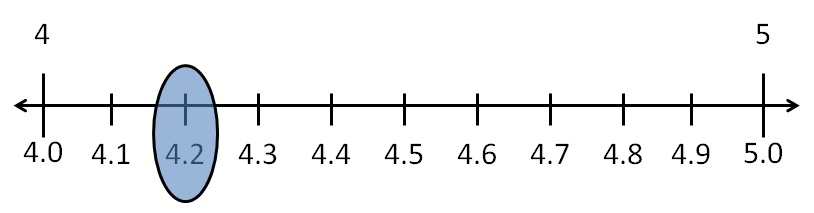Draw 28.7 on number line

Now,

28.7 > 28

&  28.7 < 29

So, 28.7 lies between 28 & 29

We draw number line from 28 & 29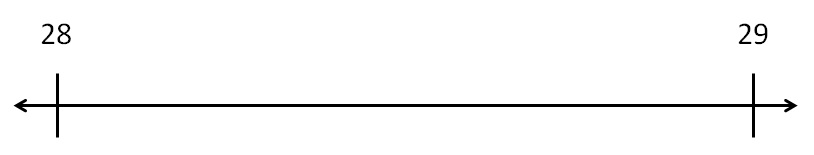We divide the number line between 28 & 29 in to 10 equal parts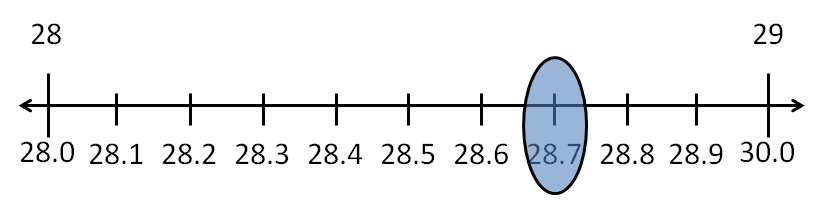#### Decimals in number line - 2 decimal point

For numbers with 2 decimal point like

2.83

We draw it in the number line

Now,

2.83 > 2

&       2.83 < 3

So, we draw number line from 2 to 3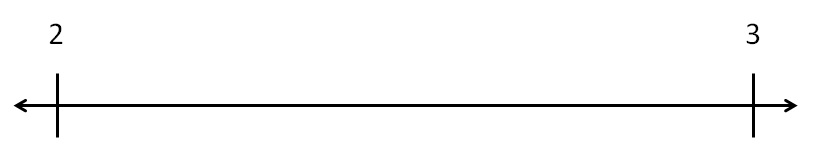We divide number line between 2 to 3 in 10 equal parts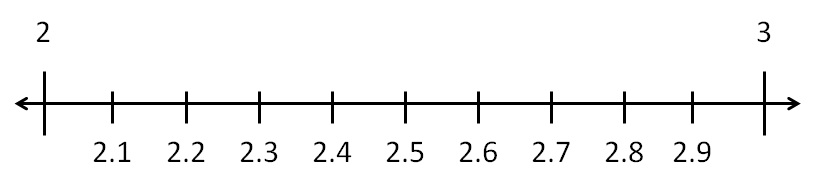Now, 2.83 will lie between 2.8 & 2.9

We divide it into 10 equal parts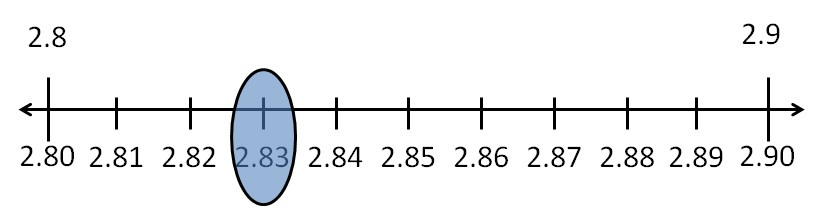#### Draw 48.85 in number line

Now,

48.85 > 48.8

&       48.85 < 48.9

So, we draw number line from 48.8 to 48.9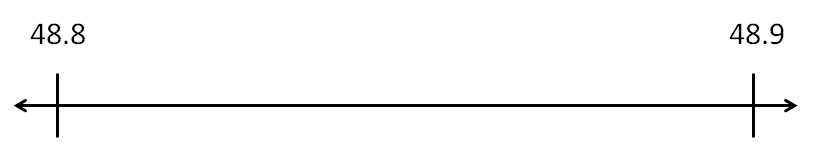We divide the number line between 48.8 & 48.9 into 10 equal parts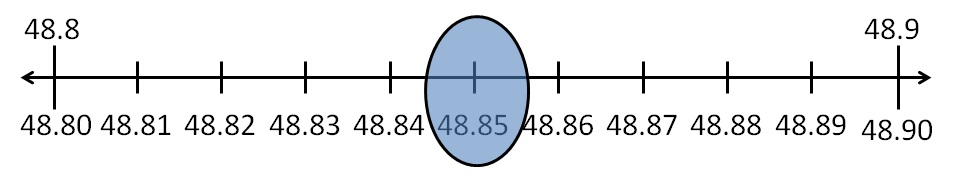Learn in your speed, with individual attention - Teachoo Maths 1-on-1 Class